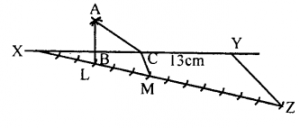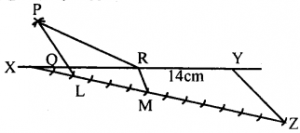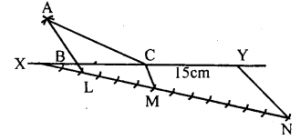# KSEEB Solutions for Class 8 Maths Chapter 12 Construction of Triangles Ex 12.7

Students can Download Maths Chapter 12 Construction of Triangles Ex 12.7 Questions and Answers, Notes Pdf, KSEEB Solutions for Class 8 Maths helps you to revise the complete Karnataka State Board Syllabus and score more marks in your examinations.

## Karnataka Board Class 8 Maths Chapter 12 Construction of Triangles Ex 12.7

Question 1.
Construct a triangle ABC whose perimeter is 13cm and whose sides are in the ratio 3 : 4 : 5.
3 + 4 + 5 = 12Question 2.
Construct a triangle PQR whose perimeter is 14 cm and whose sides are in the ratio 2 : 4 : 5.